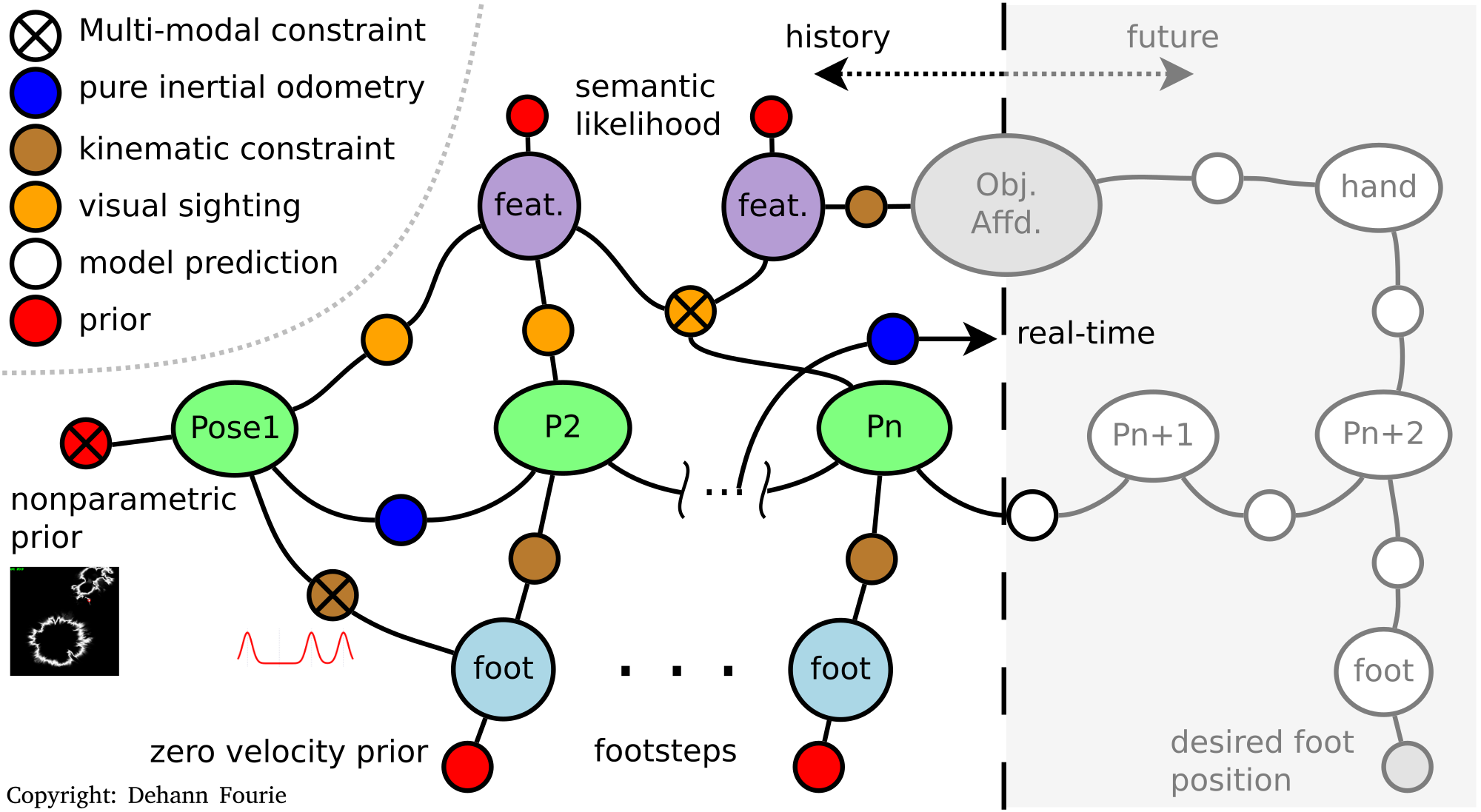Caesar Concepts

Caesar Concepts

A factor graph is a bipartite representation where variables (denoted by larger nodes) are interconnected by a set of factors (smaller nodes) that represent some algebraic interaction between the variables. Factors must adhere to the limits of probabilistic models – for example conditional likelihoods (between multiple variables) or priors (unary to one variable). A more heterogeneous factor graph example is shown below, and a broader discussion here (author disclosure):.

What are Variables and Factors

Factor graphs are bipartite, i.e. variables and factors. In practice we use "nodes" to represent both variables and factors with edges between. In future, we will remove the wording "node" from anything Factor Graph usage/abstraction related (only vars and factors). Nodes and edges will be used as terminology for actually storing the data on some graph storage/process foundation technology.

Even more meta – factors are "variables" that have already been observed and are now stochastically "fixed". Waving hands over the fact that a factors encode both the algebraic model AND the observed measurement values.

Variables in the factor graph have not been observed, but we want to back them out from the observed values and algebra relating them all. If factors are constructed from statistically independent measurements (i.e. no direct correlations between measurements other than the algebra already connecting them), then we can use Probabilistic Chain rule to write inference operation down (unnormalized):

\$P(VAR | MEAS) = P(MEAS | VAR) P(VAR)\$

where

\$P(VAR , MEAS) = P(MEAS | VAR) P(VAR)\$,

or

\$P(VAR, MEAS) = P(VAR | MEAS) P(MEAS)\$

You'll notice the first looks like "Bayes rule" and we take \$P(MEAS)\$ as a constant (the uncorrelated assumption).

Getting Started with Caesar

This section discusses the various concepts in the Caesar framework.

The initial steps in constructing and solving graphs can be found in Building and Solving Graphs.

We also recommend reviewing the various examples available in the Examples section.

Visualization

Caesar supports various visualizations and plots by using Arena, RoMEPlotting, and Director. This is discussed in Visualization with Arena.jl and RoMEPlotting.jl

Extending Caesar

The variables and factors in Caesar should be sufficient for the majority of robotic applications, however Caesar allows users to extend the framework without changing the core code. This is discussed in Creating New Variables and Factors.

Connectivity and Extensibility

Caesar supports both in-memory solving (really fast, but for moderately-sized graphs) as well as database-driven solving (think massive graphs and multiple sessions). This is still under development/being refactored, and is discussed in Common Data Persistence and Inference.

Although Caesar is Julia-based, it provides multi-language support with a ZMQ interface. This is discussed in Caesar Multi-Language Support.